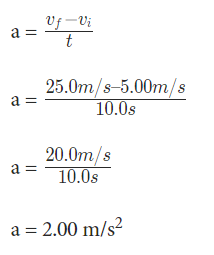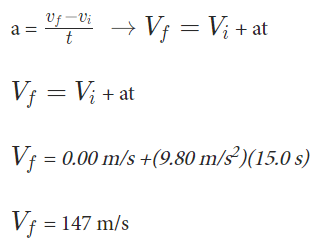# Acceleration Formula: Definition, Speed, Solved Examples

You must have heard of the term acceleration in your daily life. If we look at it in general, acceleration is said to be when an object is increasing its speed cautiously. In other words, it means if you are traveling in a car which is moving at the speed of 60 kmph and after 1 min the speed of the car is 65 kmph, which means you are accelerating. Now the question arises that how can you say that this object is accelerating? What are the terms taken into consideration during the calculation of acceleration? We will dive deeper below and learn the acceleration formula.

## Acceleration Formula | Definition

In general, we can say acceleration refers to speeding up. However, from the physics perspective, we see it means something different. Over here, it is referred to as the rate at which the velocity of an object changes. It does not matter if it is speeding up or down, it’s the change.

Therefore, the acceleration is positive when the object is speeding up and negative when speeding down. According to Newton’s Second Law, the net unbalanced force which acts on the object causes this to happen. Thus, it can be said that acceleration is a vector quantity. It is so because it changes the time rate of change of velocity.

### Formula for Acceleration

There are two formulas for acceleration. The first formula is from Newton’s second law relates force, mass, and acceleration in an equation. Thus, the formula is:

F= ma

Over here:

F refers to the force

m is the mass

a is the acceleration

Further, we have another formula that is made to calculate the rate of change in velocity over the period of time. Therefore, the formula for this is:### Solved Examples on Acccerelation Formula

Question- A woman is traveling by her sports car at a constant velocity v = 5.00 m/s. When she steps on the gas, it makes the car to accelerate forward. Further, past 10.0 seconds, she stops the acceleration and continues a constant velocity v = 25.0 m/s. Calculate the acceleration of the car.

Answer- In the forward direction, initial velocity isTherefore, we see that the acceleration of the car is 2.00 m/sforward.

Question- A man takes a rock and drops it off from a cliff. It falls for 15.0 s before it hits the ground. The acceleration due to gravity g = 9.80 m/s2. Calculate the velocity of the rock the moment before it had hit the ground.

Answer- The man released the rock from rest, therefore, we get the initial velocity as

$V_{i}$

= 0.00 m/s. The time for the change to take place is 15.0 s. The acceleration for this is 9.80 m/s2. Therefore, to find the velocity we will rearrange the equation like:Therefore, as the rock is falling, the direction of the velocity is down.